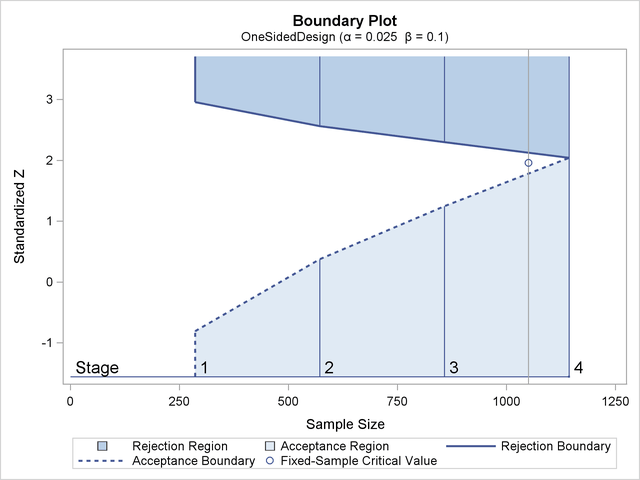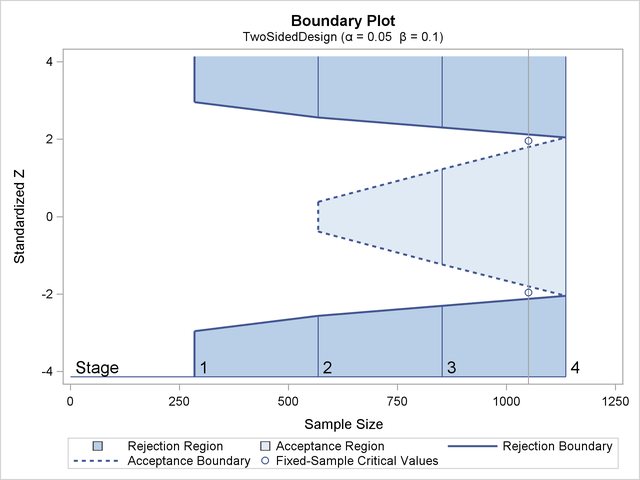### Boundary Variables

The boundaries created in group sequential trials depend on the type of the alternative hypothesis and the early stopping criterion. Table 83.5 shows the boundaries created with various design specifications.

Table 83.5: Boundary Variables

Specifications

Boundary Variables

Alternative

Lower

Upper

Hypothesis

Early Stopping

Alpha

Beta

Beta

Alpha

Lower

AcceptX

RejectX

Accept/RejectX

X

Upper

AcceptX

RejectX

Accept/RejectX

X

Two-sided

AcceptX

X

RejectX

X

Accept/RejectX

X

X

X

Up to four boundaries can be generated in a group sequential design:

• the upperboundary, to reject the null hypothesis for the upper alternative

• the upperboundary, to accept the null hypothesis with an upper alternative

• the lowerboundary, to accept the null hypothesis with a lower alternative

• the lowerboundary, to reject the null hypothesis for the lower alternative

For a two-sided design, the null hypothesis is accepted only if both the hypothesis is accepted with an upper alternative and the hypothesis is accepted with a lower alternative.

For a one-sided design with a lower alternative, only the lower boundaries are created. Similarly, for a one-sided design with an upper alternative, only the upper boundaries are created. For example, Figure 83.10 shows the boundary plot for a one-sided test with an upper alternative.

Figure 83.10: Boundary Plot for One-Sided TestFigure 83.10 corresponds to a one-sided sequential design with early stopping to reject or accept the null hypothesis. For a sequential test with early stopping only to reject the null hypothesis, there are no acceptance boundary values at interim stages. The acceptance boundary value and its associated acceptance region are displayed only at the final stage. Similarly, for a sequential test with early stopping only to accept the null hypothesis, there are no rejection boundary values at interim stages. The rejection boundary value and its associated rejection region are displayed only at the final stage.

For a two-sided design, both the lower and upper boundaries are created. For a design with early stopping to reject the null hypothesis,boundaries are created. Similarly, for a design with early stopping to accept the null hypothesis,boundaries are created. For a design with early stopping to accept or reject the null hypothesis, both theandboundaries are created.

For example, Figure 83.11 shows the boundary plot for a two-sided test.

Figure 83.11: Boundary Plot for Two-Sided TestFigure 83.11 corresponds to a two-sided sequential design with early stopping to reject or accept the null hypothesis. For a sequential test with early stopping only to reject the null hypothesis, there are no acceptance boundary values at interim stages. The acceptance boundary value and its associated acceptance region are displayed only at the final stage. Similarly, for a sequential test with early stopping only to accept the null hypothesis, there are no rejection boundary values at interim stages. The rejection boundary value and its associated rejection region are displayed only at the final stage.